# Proof of the Ratio Test

Previous: Absolute Convergence Implies Convergence

Next: List of Videos in the ISM

We can now provide the proof of the ratio test. Recall the ratio test:

The Ratio Test
To apply the ratio test to a given infinite serieswe evaluate the limit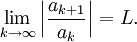There are three possibilities:

1. if L < 1, then the series converges
2. if L > 1, then the series diverges
3. if L = 1, then the test is inconclusive

## Proof

Our proof will be in two parts:

1. Proof of 1 (if L < 1, then the series converges)
2. Proof of 2 (if L > 1, then the series diverges)

### Proof of 1 (if L < 1, then the series converges)

Our aim here is to compare the given serieswith a convergent geometric series (we will be using a comparison test).

In this first case, L is less than 1, so we may choose any number r such that L < r < 1. Since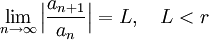the ratio |an+1/an| will eventually be less than r. In other words, there exists an integer N such thatThis follows from the formal definition of limit, which not all calculus students have covered. Those students who have not covered a formal definition of limit may wish to consult a calculus textbook on this (any of the textbooks listed on the Recommended Resources page cover it).

We can rearranged our expression to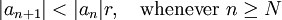If we letequal N, N + 1, N + 2 in the previous equation we obtain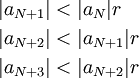and, in general,Then the series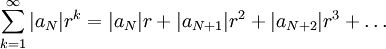is convergent because it is a geometric series with a common ratio r, such that 0 < r < 1. By the Comparison Test, the seriesis convergent, and so our given seriesis also convergent (adding a finite number of finite terms to a convergent series will create another convergent series).

Therefore, our series is absolutely convergent (and therefore convergent).

### Proof of 2 (if L > 1, then the series diverges)

Our aim here is to show that the series must be divergent using the Divergence Test.

If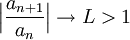then the ratiowill eventually be greater than 1; that is, there exists an integer N such thatThis means |an+1| > |an| whenever nN, and so by the definition of limitTherefore, the given series diverges by the Divergence Test.

Previous: Absolute Convergence Implies Convergence

Next: List of Videos in the ISM